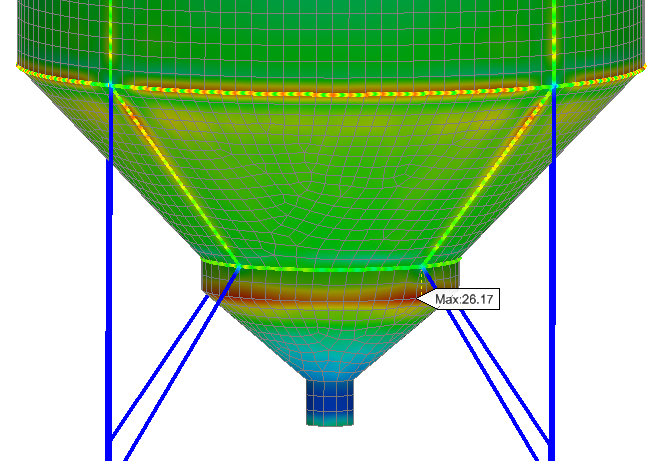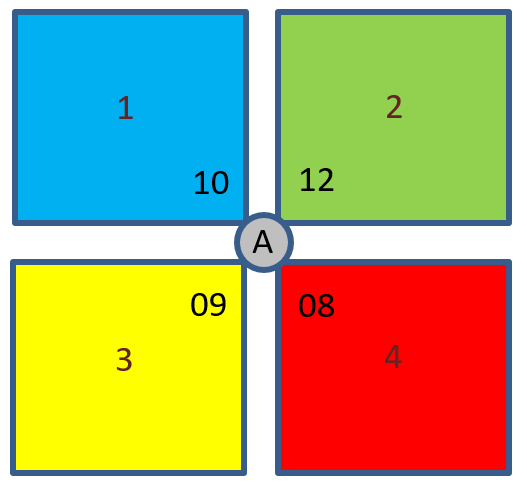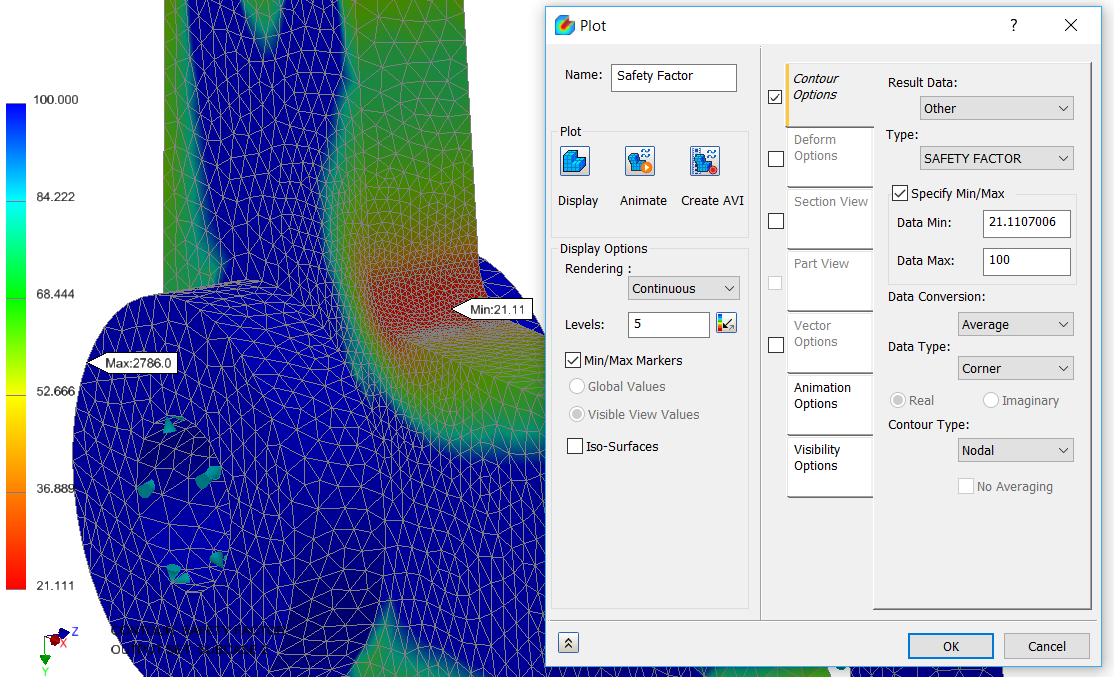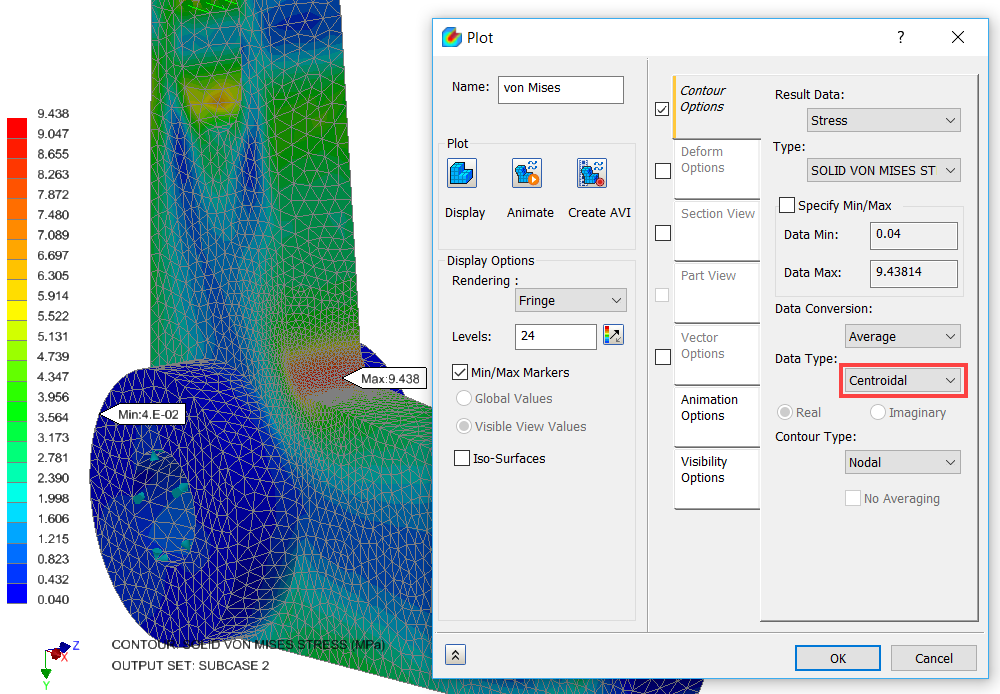# Simulation for Designers - Part 7### Simulation for Designers - Part 7

Safety Factor Plots

I frequently get told that safety factor plots results within Nastran In-CAD are not correct. The reason for this is that safety factor values are calculated in a slightly different way.

This is best explained by the following example. I will use 200MPa for yield limit for comparison purposes

Safety factor calculation based on average stress at location A is 20.51Average Stress value = (10+12+9+8)/4 = 9.75

Safety Factor = 200/9.75 = 20.51

Safety factor calculation based on how Nastran In-CAD displays plots at Location A is 20.97.

Safety Factor for Element 1 = 200/10 = 20

Safety Factor for Element 2 = 200/12 = 16.667

Safety Factor for Element 3 = 200/9 = 22.222

Safety Factor for Element 4 = 200/8 = 25

Average Safety Factor = (20+16.667+22.222+25)/4 = 20.97

So, this is the reason why there is slight difference in the safety factor results. Both results are correct as they have been calculated in slightly different ways. It is entirely up to you which value you take.Here a safety factor display is showing a minimum value of 21.11.

Note changing Data Type to Corner or Centroidal will yield the same safety factor result.

So, for the same model and results if we display the average centroidal results the maximum stress value becomes 9.4381MPa.

So, based on this value the factor of safety (hand calculation) is 21.19.This is very close to the Safety Factor plot value of 21.11 with a difference of less than 0.5%.

There is also a great article on “Safety factor is not correctly reconciled in Autodesk Nastran In-CAD” by John Holtz from Autodesk which you may find very useful. (Available here)

NB: If you cannot wait and would like to learn more about Nastran In-CAD now then I would recommend the 'Up and Running with Autodesk Nastran In-CAD 2019' book available from Amazon worldwide.

If you would to watch some of the videos I have created around Autodesk Simulation then please take a look at our Simulation webinar library.

Blog

### Inventor PDF creation with Autodesk Vault - How Sovelia Vault adds value and quality to your work?

07 September 2021

Autodesk Vault can produce PDF files from drawings, Inventor Drawings and AutoCAD drawings, and so can Sovelia Vault. This blog post focuses on the added value and the benefits of using Sovelia Vault with your Autodesk software.

Blog